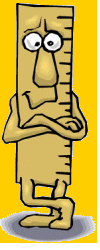Saturday 21st September 2019# Teacher Notes

KS2 Mathematics - Using and applying shape space and measures - 1a and 1f

These are aimed at consolidating the application and facts of the units of measure of capacity, mass and length, as well as some basic conversions of metric units. Children need type in the correct answer.

 Converting Units of Length - 1There are 1000 metres (m) in every kilometre (km)There are 100 centimetres (cm) in every metre(m)There are 10 millimetres (mm) in every centimetre (cm)TYPE in the correct answer for each of these conversions.1) 1000m km          2) 1500m km          3) 2800m km4) 1km m          5) 1.2km m          6) 0.5km m7) 1m cm          8) 1.25m cm          9) 2.78m cm